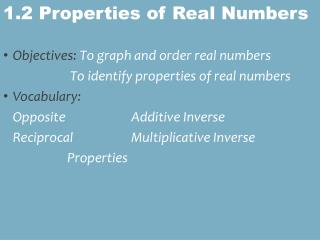# 1.2 Properties of Real Numbers - PowerPoint PPT PresentationDownload Presentation1.2 Properties of Real Numbers

1.2 Properties of Real NumbersDownload Presentation## 1.2 Properties of Real Numbers

- - - - - - - - - - - - - - - - - - - - - - - - - - - E N D - - - - - - - - - - - - - - - - - - - - - - - - - - -
##### Presentation Transcript

1. Objectives: To graph and order real numbers To identify properties of real numbers • Vocabulary: Opposite Additive Inverse Reciprocal Multiplicative Inverse Properties 1.2 Properties of Real Numbers

2. Vocabulary Terms: Properties of Real NumbersLet a, b, and c represent real numbers

3. Real Number Classifications Subsets of the Real Numbers Rational Integers Irrational Π, Whole Natural

4. Use natural numbers to count - {1,2,3,4,5,6….} • The whole numbers are the natural numbers plus 0 - {0,1,2,3….} • Integers are the natural numbers and their opposites plus 0 - {...,-3,-2,-1,0,1,2,3…} • Rational numbers are all numbers that can be written as a quotient of integers. a/b, b≠0. • Rational numbers include terminating decimals…1/8 = .0125 • Rational Numbers include repeating decimals… • 1/3 = .3333333333333333333333333333333333, or 0. with a hat over it Examples

5. CLASSIFY EACH NUMBER name ALL sets to which each belongs -1 3 √17 ⅜ 0 -5.555 • real, rational, integer • real, rational, integer, whole, natural • real, irrational • real, rational • real, rational, integer, whole • real, rational

6. Properties of Real Numbers Commutative • Think… commuting to school. • Deals with ORDER. It doesn’t matter what order you ADD or MULTIPLY. • a+b = b+a • 4 • 6 = 6 • 4

7. Properties of Real NumbersAssociative • Think…the people you associate with; your group. Are you the member of more than 1 club? • Deals with grouping when you Add or Multiply. • Order does not change. • Additive (a + b) + c = a + ( b + c) • Multiplicative (nm)p = n(mp)

8. Properties of Real NumbersIdentity Additive Identity Property • s + 0 = s • 0 is the additive identity. Multiplicative Identity Property • 1(b) = b • 1 is the multiplicative identity

9. Properties of Real Numbersinverse • Multiplicative Inverse Property • Product = 1 • a ∙ 1/a = 1, a ≠ 0 • 8(1/8) = 1 • -5(-1/5)=1 • Additive Inverse Property • Sum = Zero • a + (-a) = 0 • 12 + (− 12 ) = 0 • −7 + 7 = 0

10. Properties of Real Numbers Distributive Distributive Property • a(b + c) = ab + ac • 9(r + s) = 9r + 9s

11. Name the Property • 5 = 5 + 0 • 5(2x + 7) =10x + 35 • 8 • 7 = 7 • 8 • 24(2) = 2(24) • (7 + 8) + 2 =2 + (7 + 8)

12. Name the Property Additive Identity Distributive Commutative Commutative Commutative • 5 = 5 + 0 • 5(2x + 7) =10x + 35 • 8 • 7 = 7 • 8 • 24(2) = 2(24) • (7 + 8) + 2 =2 + (7 + 8)

13. Name the Property • 7 + (8 + 2) = (7 + 8) + 2 • 1 • v + -4 = v + -4 • (6 - 3a)b = 6b - 3ab • 4(a + b) = 4a + 4b

14. 7 + (8 + 2) = (7 + 8) + 2 1 • v + -4 = v + -4 (6 - 3a)b = 6b - 3ab 4(a + b) = 4a + 4b Associative Multiplicative Identity Distributive Distributive Name the Property

15. Vocabulary Terms: Properties of Real NumbersLet a, b, and c represent real numbers reciprocal